# User-Defined Types in Web Services

The Web methods we have demonstrated so far all received and returned simple type values. It is also possible to process user-defined typesknown as custom typesin a Web service. These types can be passed to or returned from Web methods. Web service clients also can use these user-defined types, because the proxy class created for the client contains the type definitions.

This section presents an EquationGenerator Web service that generates random arithmetic equations of type Equation. The client is a math-tutoring application that inputs information about the mathematical question that the user wishes to attempt (addition, subtraction or multiplication) and the skill level of the user (1 specifies equations using one-digit numbers, 2 specifies equations involving two-digit numbers and 3 specifies equations containing three-digit numbers). The Web service then generates an equation consisting of random numbers with the proper number of digits. The client application receives the Equation and displays the sample question to the user in a Windows Form.

Serialization of User-Defined Types

We mentioned earlier that all types passed to and from Web services must be supported by SOAP. How, then, can SOAP support a type that is not even created yet? Custom types that are sent to or from a Web service are serialized, enabling them to be passed in XML format. This process is referred to as XML serialization.

Requirements for User-Defined Types Used with Web Methods

Classes that are used to specify return types and parameter types for Web methods must meet several requirements:

1. They must provide a public default or parameterless constructor. When a Web service or Web service consumer receives an XML serialized object, the .NET Framework must be able to call this constructor as part of the process of deserializing the object (i.e., converting it back to a C# object).
2. Properties and instance variables that should be serialized in XML format must be declared public. (Note that the public properties can be used to provide access to private instance variables.)
3. Properties that should be serialized must provide both get and set accessors (even if they have empty bodies). Read-only properties are not serialized.

Any data that is not serialized simply receives its default value (or the value provided by the default or parameterless constructor) when an object of the class is deserialized.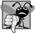## Common Programming Error 22 3

Failure to define a default or parameterless public constructor for a type being passed to or returned from a Web method is a runtime error.## Common Programming Error 22 4

Defining only the get or set accessor of a property for a user-defined type being passed to or returned from a Web method results in a property that is inaccessible to the client.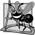## Software Engineering Observation 22 1

Clients of a Web service can access only the service's public members. The programmer can provide public properties to allow access to private data.

Defining Class Equation

We define class Equation in Fig. 22.26. Lines 2846 define a constructor that takes three argumentstwo ints representing the left and right operands and a string that represents the arithmetic operation to perform. The constructor sets the leftOperand, rightOperand and operationType instance variables, then calculates the appropriate result. The parameterless constructor (lines 2125) calls the three-argument constructor (lines 2846) and passes some default values. We do not use the parameterless constructor explicitly, but the XML serialization mechanism uses it when objects of this class are deserialized. Because we provide a constructor with parameters, we must explicitly define the parameterless constructor in this class so that objects of the class can be passed to or returned from Web methods.

Figure 22.26. Class that stores equation information.

 ``` 1 // Fig. 22.26: Equation.cs 2 // Class Equation that contains information about an equation. 3 using System; 4 using System.Data; 5 using System.Configuration; 6 using System.Web; 7 using System.Web.Security; 8 using System.Web.UI; 9 using System.Web.UI.WebControls; 10 using System.Web.UI.WebControls.WebParts; 11 using System.Web.UI.HtmlControls; 12 13 public class Equation 14 { 15 private int leftOperand; // number to the left of the operator 16 private int rightOperand; // number to the right of the operator 17 private int resultValue; // result of the operation 18 private string operationType; // type of the operation 19 20 // required default constructor 21 public Equation() 22 : this( 0, 0, "+" ) 23 { 24 // empty body 25 } // end default constructor 26 27 // three-argument constructor for class Equation 28 public Equation( int leftValue, int rightValue, string type ) 29 { 30 leftOperand = leftValue; 31 rightOperand = rightValue; 32 operationType = type; 33 34 switch ( operationType ) // perform appropriate operation 35 { ``` 36 case "+": // addition 37 resultValue = leftOperand + rightOperand; 38 break; 39 case "-": // subtraction 40 resultValue = leftOperand - rightOperand; 41 break; 42 case "*": // multiplication 43 resultValue = leftOperand * rightOperand; 44 break; 45 } // end switch 46 } // end three-argument constructor 47 48 // return string representation of the Equation object 49 public override string ToString() 50 { 51 return leftOperand.ToString() + " " + operationType + " " + 52 rightOperand.ToString() + " = " + resultValue.ToString(); 53 } // end method ToString 54 55 // property that returns a string representing left-hand side 56 public string LeftHandSide 57 { 58 get 59 { 60 return leftOperand.ToString() + " " + operationType + " " + 61 rightOperand.ToString(); 62 } // end get 63 64 set // required set accessor 65 { 66 // empty body 67 } // end set 68 } // end property LeftHandSide 69 70 // property that returns a string representing right-hand side 71 public string RightHandSide 72 { 73 get 74 { 75 return resultValue.ToString(); 76 } // end get 77 78 set // required set accessor 79 { 80 // empty body 81 } // end set 82 } // end property RightHandSide 83 84 // property to access the left operand 85 public int Left 86 { 87 get 88 { 89 return leftOperand; 90 } // end get 91 92 set 93 { 94 leftOperand = value; 95 } // end set 96 } // end property Left 97 98 // property to access the right operand 99 public int Right 100 { 101 get 102 { 103 return rightOperand; 104 } // end get 105 106 set 107 { 108 rightOperand = value; 109 } // end set 110 } // end property Right 111 112 // property to access the result of applying 113 // an operation to the left and right operands 114 public int Result 115 { 116 get 117 { 118 return resultValue; 119 } // end get 120 121 set 122 { 123 resultValue = value; 124 } // end set 125 } // end property Result 126 127 // property to access the operation 128 public string Operation 129 { 130 get 131 { 132 return operationType; 133 } // end get 134 135 set 136 { 137 operationType = value; 138 } // end set 139 } // end property Operation 140 } // end class Equation

Class Equation defines properties LeftHandSide (lines 5668), RightHandSide (lines 7182), Left (lines 8596), Right (lines 99110), Result (lines 114125) and Operation (lines 128139). The client of the Web service does not need to modify the values of properties LeftHandSide and RightHandSide. However, recall that a property can be serialized only if it has both a get and a set accessorthis is true even if the set accessor has an empty body. LeftHandSide (lines 5668) returns a string representing everything to the left of the equals (=) sign in the equation, and RightHandSide (lines 7182) returns a string representing everything to the right of the equals (=) sign. Left (lines 8596) returns the int to the left of the operator (known as the left operand), and Right (lines 99110) returns the int to the right of the operator (known as the right operand). Result (lines 114125) returns the solution to the equation, and Operation (lines 128139) returns the operator in the equation. The client in this case study does not use the RightHandSide property, but we included it in case future clients choose to use it.

Creating the EquationGenerator Web Service

Figure 22.27 presents the EquationGenerator Web service, which creates random, customized Equations. This Web service contains only method GenerateEquation (lines 1632), which takes two parametersa string representing the mathematical operation (addition, subtraction or multiplication) and an int representing the difficulty level.

Figure 22.27. Web service that generates random equations.

 ``` 1 // Fig. 22.27: Generator.cs 2 // Web Service to generate random equations based on a specified 3 // operation and difficulty level. 4 using System; 5 using System.Web; 6 using System.Web.Services; 7 using System.Web.Services.Protocols; 8 9 [ WebService( Namespace = "http://www.deitel.com/", Description = 10 "Web service that generates a math equation." ) ] 11 [ WebServiceBinding( ConformsTo = WsiProfiles.BasicProfile1_1 ) ] 12 public class Generator : System.Web.Services.WebService 13 { 14 // Method to generate a math equation 15 [ WebMethod( Description = "Method to generate a math equation." ) ] 16 public Equation GenerateEquation( string operation, int level ) 17 { 18 // find maximum and minimum number to be used 19 int maximum = Convert.ToInt32( Math.Pow( 10, level ) ); 20 int minimum = Convert.ToInt32( Math.Pow( 10, level - 1 ) ); 21 22 // object to generate random numbers 23 Random randomObject = new Random(); 24 25 // create equation consisting of two random 26 // numbers between minimum and maximum parameters 27 Equation equation = new Equation( 28 randomObject.Next( minimum, maximum ), 29 randomObject.Next( minimum, maximum ), operation ); ``` 30 31 return equation; 32 } // end method GenerateEquation 33 } // end class Generator

Testing the EquationGenerator Web Service

Figure 22.28 shows the result of testing the EquationGenerator Web service. Note that the return value from our Web method is XML-encoded. However, this example differs from previous ones in that the XML specifies the values for all public properties and data of the object that is being returned. The return object has been serialized in XML. Our proxy class takes this return value and deserializes it into an object of class Equation, then passes it to the client.

Figure 22.28. Returning an XML serialized object from a Web method.

a) Invoking the GenerateEquation method to create a subtraction equation with two-digit numbers.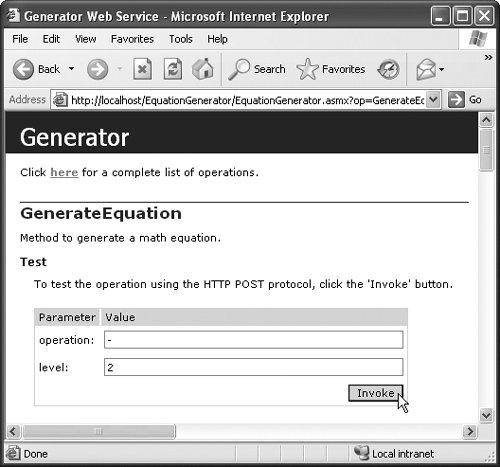b) XML encoded results of invoking the GenerateEquation method to create a subtraction equation with two-digit numbers.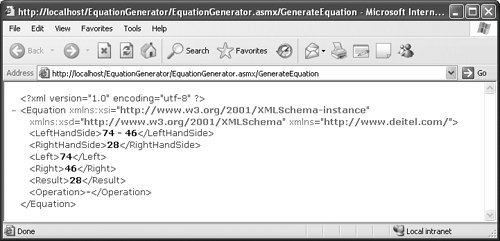Note that an Equation object is not being passed between the Web service and the client. Rather, the information in the object is being sent as XML-encoded data. Clients created using .NET will take the information and create a new Equation object. Clients created on other platforms, however, may use the information differently. Readers creating clients on other platforms should check the Web services documentation for the specific platform they are using, to see how their clients may process custom types.

Let's examine Web method GenerateEquation more closely. Lines 1920 of Fig. 22.27 define the upper and lower bounds for the random numbers that the method uses to generate an Equation. To set these limits, the program first calls static method Pow of class Maththis method raises its first argument to the power of its second argument. To calculate the value of maximum (the upper bound for any randomly generated numbers used to form an Equation), the program raises 10 to the power of the specified level argument (line 19). If level is 1, maximum is 10; if level is 2, maximum is 100; and if level is 3, maximum is 1000. Variable minimum's value is determined by raising 10 to a power one less than level (line 20). This calculates the smallest number with level digits. If level is 1, minimum is 1; if level is 2, minimum is 10; and if level is 3, minimum is 100.

Lines 2729 create a new Equation object. The program calls Random method Next, which returns an int that is greater than or equal to the specified lower bound, but less than the specified upper bound. This method generates a left operand value that is greater than or equal to minimum but less than maximum (i.e., a number with level digits). The right operand is another random number with the same characteristics. Line 29 passes the string operation received by GenerateEquation to the Equation constructor. Line 31 returns the new Equation object to the client.

Consuming the EquationGenerator Web Service

The MathTutor application (Fig. 22.29) uses the EquationGenerator Web service. The application calls the Web service's GenerateEquation method to create an Equation object. The tutor then displays the left-hand side of the Equation and waits for user input. This example accesses classes Generator and Equation from the localhost namespaceboth are placed in this namespace by default when the proxy is generated. We declare variables of these types at lines 2223. Line 23 also creates the Generator proxy.

Figure 22.29. Math-tutoring application.

 ``` 1 // Fig. 22.29: MathTutor.cs 2 // Math tutoring program using Web service to generate random equations. 3 using System; 4 using System.Collections.Generic; 5 using System.ComponentModel; 6 using System.Data; 7 using System.Drawing; 8 using System.Text; 9 using System.Windows.Forms; 10 11 namespace MathTutor 12 { 13 public partial class MathTutorForm : Form 14 { 15 public MathTutorForm() 16 { ``` 17 InitializeComponent(); 18 } // end constructor 19 20 private string operation = "+"; 21 private int level = 1; 22 private localhost.Equation equation; 23 private localhost.Generator generator = new localhost.Generator(); 24 25 // generates new equation when user clicks button 26 private void generateButton_Click( object sender, EventArgs e ) 27 { 28 // generate equation using current operation and level 29 equation = generator.GenerateEquation( operation, level ); 30 31 // display left-hand side of equation 32 questionLabel.Text = equation.LeftHandSide; 33 34 okButton.Enabled = true; 35 answerTextBox.Enabled = true; 36 } // end method generateButton_Click 37 38 // check user's answer 39 private void okButton_Click( object sender, EventArgs e ) 40 { 41 // determine correct result from Equation object 42 int answer = equation.Result; 43 44 if ( answerTextBox.Text == "" ) 45 return; 46 47 // get user's answer 48 int userAnswer = Int32.Parse( answerTextBox.Text ); 49 50 // determine whether user's answer is correct 51 if ( answer == userAnswer ) 52 { 53 questionLabel.Text = ""; // clear question 54 answerTextBox.Text = ""; // clear answer 55 okButton.Enabled = false; // disable OK button 56 MessageBox.Show( "Correct! Good job!" ); 57 } // end if 58 else 59 MessageBox.Show( "Incorrect. Try again." ); 60 } // end method okButton_Click 61 62 // set difficulty level to 1 63 private void levelOneRadioButton_CheckedChanged( object sender, 64 EventArgs e ) 65 { 66 level = 1; 67 } // end method levelOneRadioButton_CheckedChanged 68 69 // set difficulty level to 2 70 private void levelTwoRadioButton_CheckedChanged( object sender, 71 EventArgs e ) 72 { 73 level = 2; 74 } // end method levelTwoRadioButton_CheckedChanged 75 76 // set difficulty level to 3 77 private void levelThreeRadioButton_CheckedChanged( object sender, 78 EventArgs e ) 79 { 80 level = 3; 81 } // end method levelThreeRadioButton_CheckedChanged 82 83 // set the operation to addition 84 private void additionRadioButton_CheckedChanged( object sender, 85 EventArgs e ) 86 { 87 operation = "+"; 88 generateButton.Text = 89 "Generate " + additionRadioButton.Text + " Example"; 90 } // end method additionRadioButton_CheckedChanged 91 92 // set the operation to subtraction 93 private void subtractionRadioButton_CheckedChanged( object sender, 94 EventArgs e ) 95 { 96 operation = "-"; 97 generateButton.Text = "Generate " + 98 subtractionRadioButton.Text + " Example"; 99 } // end method subtractionRadioButton_CheckedChanged 100 101 // set the operation to multiplication 102 private void multiplicationRadioButton_CheckedChanged( 103 object sender, EventArgs e ) 104 { 105 operation = "*"; 106 generateButton.Text = "Generate " + 107 multiplicationRadioButton.Text + " Example"; 108 } // end method multiplicationRadioButton_CheckedChanged 109 } // end class MathTutorForm 110 } // end namespace MathTutor a) Generating a level 1 addition equation.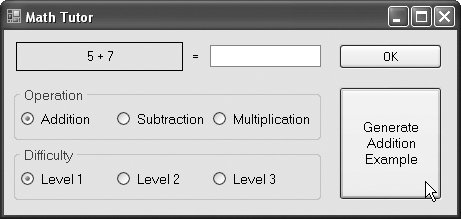b) Answering the equation incorrectly.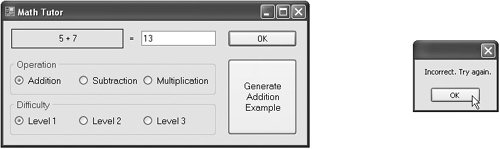c) Answering the equation correctly.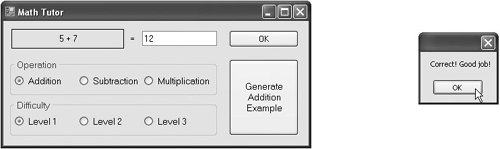d) Generating a level 2 multiplication equation.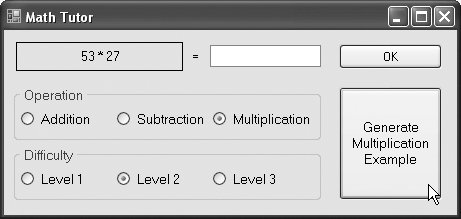The math-tutoring application displays an equation and waits for the user to enter an answer. The default setting for the difficulty level is 1, but the user can change this by choosing a level from the RadioButtons in the GroupBox labeled Difficulty. Clicking any of the levels invokes the corresponding RadioButton's CheckedChanged event handler (lines 6381), which sets integer level to the level selected by the user. Although the default setting for the question type is Addition, the user also can change this by selecting one of the RadioButtons in the GroupBox labeled Operation. Doing so invokes the corresponding operation's event handlers in lines 84108, which assigns to string operation the symbol corresponding to the user's selection. Each event handler also updates the Text property of the Generate button to match the newly selected operation.

Event handler generateButton_Click (lines 2636) invokes EquationGenerator method GenerateEquation (line 29). After receiving an Equation object from the Web service, the handler displays the left-hand side of the equation in questionLabel (line 32) and enables OkButton so that the user can enter an answer. When the user clicks OK, OkButton_Click (lines 3960) checks whether the user provided the correct answer.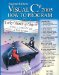Visual C# 2005 How to Program (2nd Edition)
ISBN: 0131525239
EAN: 2147483647
Year: 2004
Pages: 600